# Homology of torus

This article describes the value (and the process used to compute it) of some homotopy invariant(s) for a topological space or family of topological spaces. The invariant is homology and the topological space/family is torus
Get more specific information about torus | Get more computations of homology

## Statement

We denote by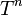$T^n$ the$n$-dimensional torus, which is the topological space:$(S^1)^n \cong S^1 \times S^1 \times \dots S^1$

i.e., the product of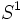$S^1$, the circle, with itself$n$ times. It is equipped with the product topology. If we think of$S^1$ as a group,$T^n$ gets a group structure too as the external direct product.

### Unreduced version over integers

The$k^{th}$ homology group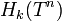$H_k(T^n)$ is a free abelian group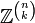$\mathbb{Z}^{\binom{n}{k}}$ of rank$\binom{n}{k}$, where$\binom{n}{k}$ denotes the binomial coefficient, or the number of subsets of size$k$ in a set of size$n$. In particular,$\binom{n}{k}$ is positive for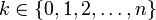$k \in \{ 0,1,2,\dots,n \}$ and zero for other$k$. Thus,$H_k(T^n)$ is nontrivial for$0 \le k \le n$ and zero for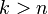$k > n$.

### Reduced version over integers

The$k^{th}$ reduced homology group$\tilde{H}_k(T^n)$ is a free abelian group of rank$\binom{n}{k}$ for$k > 0$ and is trivial for$k = 0$. In particular, it is a nontrivial group for$k \in \{ 1,2,\dots, n \}$ and is zero for other$k$.

### Unreduced version over an abelian group

The$k^{th}$ homology group$H_k(T^n;M)$ is a direct sum$M^{\binom{n}{k}}$ of rank$\binom{n}{k}$, where$\binom{n}{k}$ denotes the binomial coefficient, or the number of subsets of size$k$ in a set of size$n$. The behavior is qualitatively the same as over the integers. Note that this result is the same regardless of whether we think of the homology with coefficients in$M$ as an abelian group or as a module over some other commutative unital ring.

### Reduced version over an abelian group

This is the same as the unreduced version, except that the zeroth homology group is zero.

## Homology groups in tabular form

Below are given the ranks of homology groups for small values of$n$ and$k$. Each row corresponds to a value of$n$ and each column corresponds to a value of$k$ for which we are computing$H_k(T^n)$. If a cell value reads 2, for instance, that means that the corresponding homology group with coefficients in the integers is$\mathbb{Z}^2 = \mathbb{Z} \oplus \mathbb{Z}$ and the corresponding homology group with coefficients in a module$M^2$.[/itex] Note that the cell values for$k > n$ are omitted, because all these values are zero:$n$ Torus$T^n$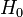$H_0$ rank$H_1$ rank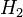$H_2$ rank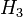$H_3$ rank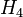$H_4$ rank$H_5$ rank
0 one-point space 1
1 circle 1 1
2 2-torus 1 2 1
3 3-torus 1 3 3 1
4 4-torus 1 4 6 4 1
5 5-torus 1 5 10 10 5 1

## Related invariants

These are all invariants that can be computed in terms of the homology groups.

Invariant General description Description of value for torus Comment
Betti numbers The$k^{th}$ Betti number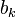$b_k$ is the rank of the$k^{th}$ homology group.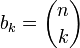$b_k = \binom{n}{k}$
Poincare polynomial Generating polynomial for Betti numbers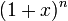$(1 + x)^n$
Euler characteristic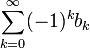$\sum_{k=0}^\infty (-1)^k b_k$ 0 (hence it is a space with Euler characteristic zero) This can also be seen from the fact that we have a group (see Euler characteristic of compact connected nontrivial Lie group is zero) or from the fact that it is a product of circles, and the Euler characteristic of the circle is zero (see Euler characteristic of product is product of Euler characteristics).